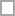# Discrete Mathematics Quizzes & Trivia

Curious and eager to learn new trivia about life, the universe, and everything? If yes, what better way to take some awesome discrete mathematics quizzes online? Test yourself and share these discrete mathematics quizzes to find out who is the quiz champ!

Enhance your knowledge about a topic or learn something completely new by answering ultimate discrete mathematics quiz questions.

Each and every discrete mathematics quiz that we have is made up of well-researched and interesting quiz questions. With detailed instant feedback for quiz answers, you can easily learn something new about discrete mathematics with every question you attempt.

Prepared by, R VISVANATHAN, PG ASST IN MATHS, GHSS, PERIYATHACHUR, TINDIVANAM...

Questions: 20  |  Attempts: 130   |  Last updated: Dec 9, 2013
• Sample Question
Which of the following are statements? (i)  May God bless you.    (ii)  Rose is a flower. (iii) Milk is white.     (iv)  1 is a prime number (1)  (i), (ii), (iii)    (2)  (i), (ii), (iv)   (3)  (i), (iii), (iv)  (4)  (ii), (iii), (iv)Ch 9 discrete mathematics quiz (9.1 - 9.4)

Questions: 20  |  Attempts: 62   |  Last updated: Jan 25, 2013

Welcome To Discrete Mathematics QuizDesigned By Layth AdeebCihan University / Faculty of Computer Science / First Semester

Questions: 6  |  Attempts: 33   |  Last updated: Jan 9, 2015
• Sample Question
"Baghdad is the Capital of Iraq"  choose all that applyShow your truth tables as solutions in a separate sheet.

Questions: 9  |  Attempts: 31   |  Last updated: Jan 19, 2018
• Sample Question
P → q is logically equivalent toMultilple Choice

Questions: 42  |  Attempts: 31   |  Last updated: Jul 30, 2011
• Sample Question
Consists of 2 finite sets: vertices and edges with a set of 1 or 2 endpointsRelated

## Discrete Mathematics Questions and Answers

• Which of the following are statements? (i)  May God bless you.    (ii)  Rose is a flower. (iii) Milk is white.     (iv)  1 is a prime number (1)  (i), (ii), (iii)    (2)  (i), (ii), (iv)   (3)  (i), (iii), (iv)  (4)  (ii), (iii), (iv)Discrete mathematics question from

• If a compound statement is made up of three simple statements, then the number of rows in the truth table is (1)  8  (2)  6  (3)  4  (4)  2Discrete mathematics question from

• The number of rows in the truth table of  is (1)  2  (2)  4  (3)  6  (4)  8Discrete mathematics question from

• Baghdad is the Capital of Iraq  choose all that applyDiscrete mathematics question fromDiscrete mathematics question from

• x<y if and only if y>x. why we consider this statement as a proposition?Discrete mathematics question from

• p → q is logically equivalent toDiscrete mathematics question from

• p ∨ q is logically equivalent toDiscrete mathematics question from

• ¬ (p ↔ q) is logically equivalent toDiscrete mathematics question from